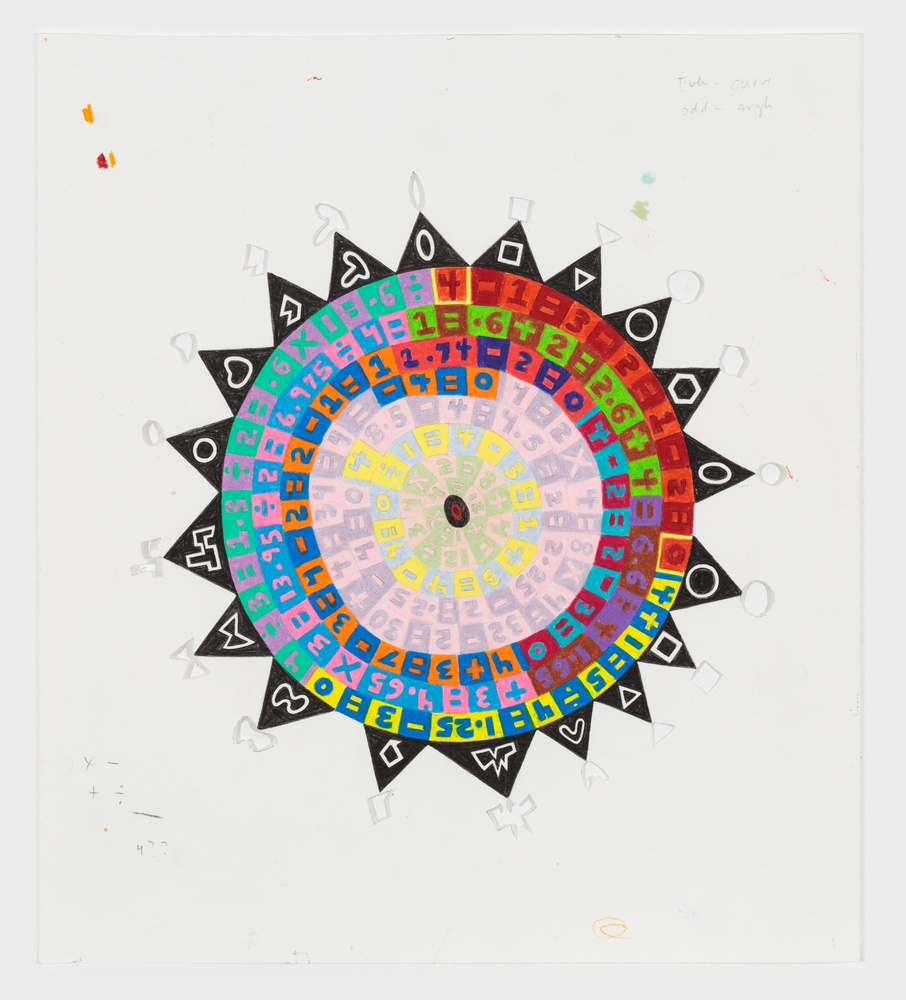#### ZERO zero

2019, Colored Pencil and Graphite on Paper

12.5 X 11 inches In this drawing, I wanted to explore the idea of entropy through basic mathematical procedures. Starting with zero, I randomly applied a mathematical function to each subsequent number, between 0 and 10. I continued this, starting at the center, until the process reached zero again, and then I started a new set. Each phase is colored differently and the shapes cut out at the edges represent a physical void.

← Back to Small Works